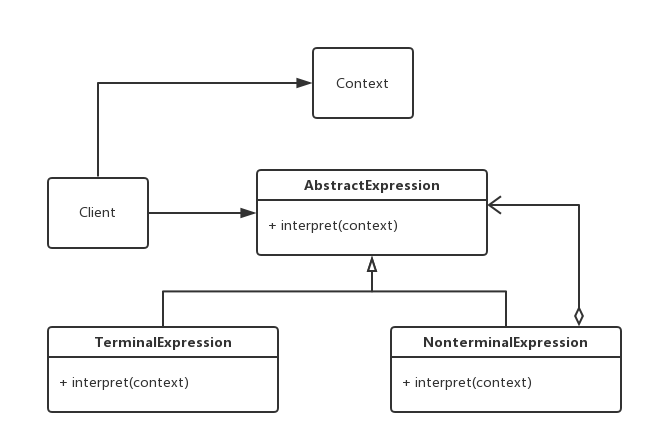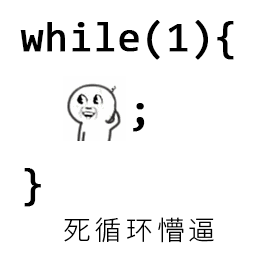# 使用场景

• 当一个语言需要解释执行，并且该语言中的句子可以表示为一个抽象的语法树时。
• 某些重复出现的问题，可以用一种简单的语言来表示的时候，可以将这个问题转化为一种语法规则下的语句。

# UML• AbstractExpression:抽象的解析方法。声明一个解析方法，具体实现在具体的子类中完成
• TernimalExprission:实现对文法中与终结符有关的解释操作。
• NonterminalExpression:实现对文法中的非终结符有关的解释操作。
• Context：包含解释器之外的全部信息。
• Client: 解析表达式，构建抽象语法书，执行具体的解释操作等。

# 简单实现

public abstract class ArithmeticExpression {
public abstract int interpret();
}

public class NumExpression extends ArithmeticExpression {
private int num;

public NumExpression(int num) {
this.num = num;
}

@Override
public int interpret() {
return num;
}
}

public abstract class OperatorExpression extends ArithmeticExpression {
protected ArithmeticExpression exp1,exp2;

public OperatorExpression(ArithmeticExpression exp1, ArithmeticExpression exp2) {
this.exp1 = exp1;
this.exp2 = exp2;
}
}

public class AddExpression extends OperatorExpression {
public AddExpression(ArithmeticExpression exp1, ArithmeticExpression exp2) {
super(exp1, exp2);
}

@Override
public int interpret() {
return exp1.interpret()+exp2.interpret();
}
}

public class Calculator {
private Stack<ArithmeticExpression> mExp = new Stack<>();
//使用的时候在构造方法里传入要计算的字符串。
public Calculator(String expression) {
//准备两个解释器
ArithmeticExpression exp1, exp2;
//将字符串按空格分割
String[] elements = expression.split(" ");
for (int i = 0; i < elements.length; i++) {
switch (elements[i].charAt(0)) {
case '+':
//如果是’+‘，说明是个运算符，将上一个数和下一个数相加，也就是当前下标i的i-1和i+1相加。
//去除栈中前一次压如的数。也就是这个加号之前的计算结果。
exp1 = mExp.pop();
//取出这个加号后面的数，解析成数字，
exp2 = new NumExpression(Integer.valueOf(elements[++i]));
//将这两个数进行计算，并将结果压入栈中。
break;
default:
//如果是数字，就解析为数字，加入栈中。
mExp.push(new NumExpression(Integer.valueOf(elements[i])));
break;
}
}
}
public int calculate(){
return mExp.pop().interpret();
}

}

public class Client {
public static void main(String[] args) {
Calculator calculator = new Calculator("1 + 2 + 3");
System.out.println(calculator.calculate());
}
}

6

public class Subtraction extends OperatorExpression {
public Subtraction(ArithmeticExpression exp1, ArithmeticExpression exp2) {
super(exp1, exp2);
}

@Override
public int interpret() {
return exp1.interpret()-exp2.interpret();
}
}

case '-':
exp1 = mExp.pop();
exp2 = new NumExpression(Integer.valueOf(elements[++i]));
mExp.push(new SubtractionExpression(exp1,exp2));
break;

public class Client {
public static void main(String[] args) {
Calculator calculator = new Calculator("1 + 2 + 3 - 1");
System.out.println(calculator.calculate());
}
}

5

# 总结

## 优点

• 拓展性很灵活，需要增加新的解释器的时候，直接实现一个新的就行了，然后在实际运用中灵活构建语法树来运用。

## 缺点

• 每一个文法都至少对应一个解释器，会产生大量的类，维护困难。
• 对于复杂的文法，要构建语法书会非常繁琐，甚至需要构建多颗语法树。